# 0.展示PTA总分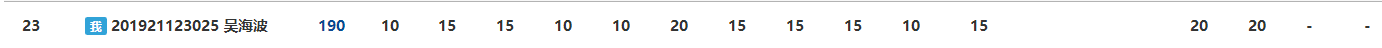# 1.本章学习总结

## 1.1学习内容总结

• 指针做循环变量：即将指针作为循环变量，在指针移动到某一个位置的时候，达到了循环结束的条件，循环结束。

for (p = a; p <= a + 9; p++)//即指针p作为循环变量 { sum = sum + *p; }

• 字符指针如何表示字符串：字符指针指向了字符数组的首地址。

定义指针*p,字符数组a[] p=a[]//字符指针表示字符数组a[]的首地址

• 动态内存分配：利用malloc或calloc（区别：malloc动态申请的内存不会自动赋初值，而calloc则会自动赋予初值0；相同点：都需要在结束时释放动态分配的内存）
if ((p = (int*)malloc(或calloc)(n * sizeof(int))) == NULL)//动态申请内存 { printf("Not able to allocate memory.\n"); exit(1); } free(p);//释放申请的内存

• 二级指针、行指针：二级指针指指向一级指针是指针，即指向地址的指针。行指针是指指针本身就指向某一行。
二级指针：int **p//二级指针
行指针：int（*p)

• 指针数组及其应用：利用指针数组存放指针地址，使用时利用指针数组中的元素来输入或者输出某地址的内容。

char* str; //开辟指针数组 for (i = 0; i < n; i++) { str[i] = (char*)malloc(10 * sizeof(char));//申请空间 scanf("%s", str[i]);//输入字符串 str=10;//利用指针数组改变某地址存储的数据 }

• 指针做函数返回值及其注意:不能在函数实现时返回函数内定义变量的地址，因为函数结束时这些对象在函数返回时就会消亡。
因此，函数指针的函数一般都返回全局数据对象或者主调函数中数据对象的地址。

## 1.2本章学习体会

• 学习较困难，在使用指针作为返回值是时常出问题。
• 大概1400行左右（反复研究教材上的题目），我个人感觉达标。

# 2.PTA实验作业

## 2.1合并两个有序数组

### 2.1.1 伪代码

定义整形flag并赋值为1 定义整形循环变量i 定义整形M,N用来暂存a,b数组的长度 while m,n都不等于0 do flag=2 if( *(a + m - 1) < *(b + n - 1)) *(a + m + n - 1) = *(b + n - 1) n减一 else if ( *(a + m - 1) > *(b + n - 1)) then *(a + m + n - 1) = *(a + m - 1) m减一 if (*(a + m - 1) = *(b + n - 1)) then *(a + m + n - 1) = *(a + m - 1); *(a + m + n - 2) = *(b + n - 1); m,n都减一 end if if(m==0且flag==2) 将b数组剩下的数字都交到a数组前端 if(m==0且flag==1) 将b数组全部直接移入a数组

### 2.1.2代码截图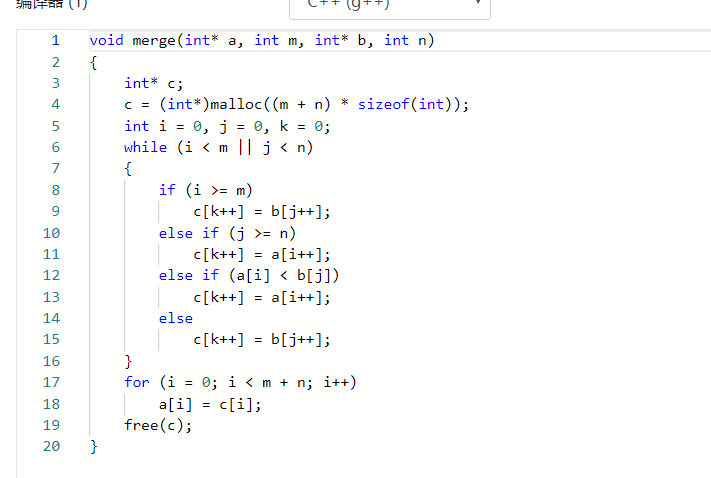### 2.1.3 总结本题的知识点

• 可以通过指针来处理数组，来改变数组某元素所存储的内容。
• 指针可以作为参数实现函数数据的传递。

### 2.1.4 PTA提交列表及说明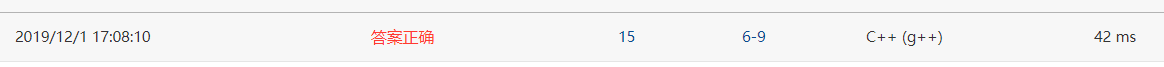## ***2.2 （指针做函数返回值） 查找指定字符

### 2.2.1 伪代码

定义len为读入字符串的长度，index初值为 - 1（只要小于0都可以） 读入需要查找的字符ch 读入字符串 for (i = 0; i < len, i++) if (找到) index=i； end for if index不等于初值 输出找到的i else 输出Not Found end if

### 2.2.2 代码截图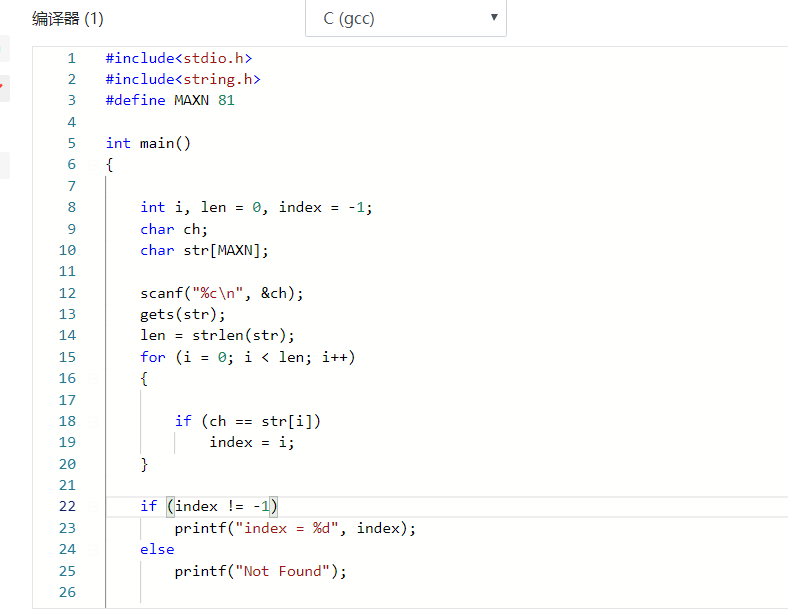### 2.2.4 PTA提交列表及说明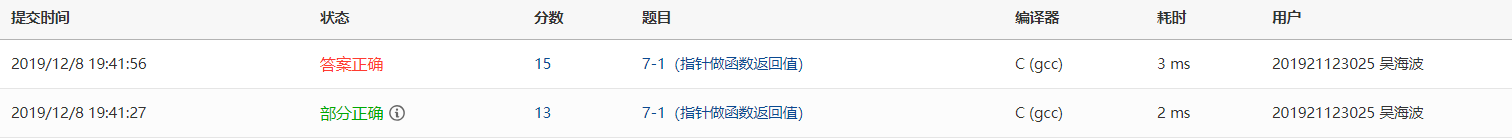## 2.3 填充矩阵

### 2.3.1 伪代码

 定义整形变量 i; 定义整形变量 j; for (i = 0; i < n; i++) for (j = 0; j < n; j++) if (i + j == n - 1) p[i][j] = 1; else if (i + j < n - 1) p[i][j] = 3; else p[i][j] = 2; end for

### 2.3.2 代码截图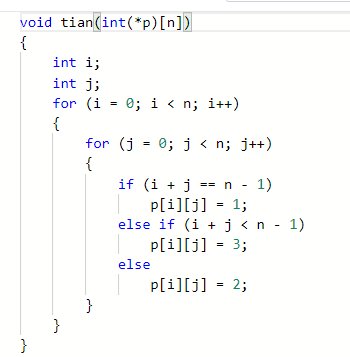### 2.2.4 PTA提交列表及说明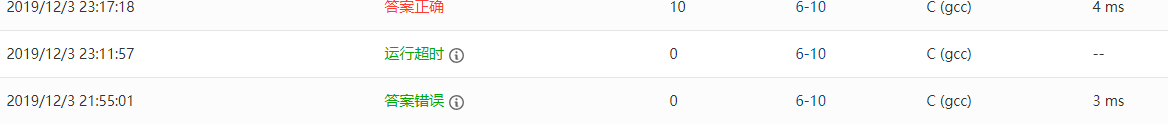# 3.阅读代码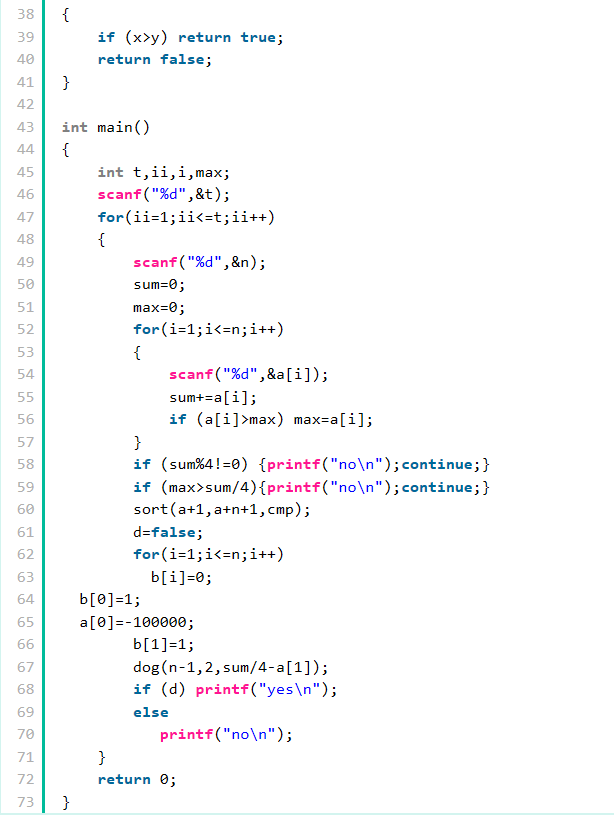posted on 2019-12-01 17:15  网1吴海波  阅读(217)  评论(0编辑  收藏  举报